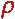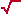Math & Science Home | Proficiency Tests | Mathematical Thinking in Physics | Aeronauts 2000

 CONTENTS Introduction Fermi's Piano Tuner Problem How Old is Old? If the Terrestrial Poles were to Melt... Sunlight Exerts Pressure Falling Eastward What if an Asteroid Hit the Earth Using a Jeep to Estimate the Energy in Gasoline How do Police Radars really work? How "Fast" is the Speed of Light? How Long is a Light Year? How Big is a Trillion? "Seeing" the Earth, Moon, and Sun to Scale Of Stars and Drops of Water If I Were to Build a Model of the Cosmos... A Number Trick Designing a High Altitude Balloon Pressure in the Vicinity of a Lunar Astronaut Space Suit due to Outgassing of Coolant Water Calendar Calculations Telling Time by the Stars - Sidereal Time Fields, an Heuristic Approach The Irrationality ofThe Irrationality ofThe Number (i)i Estimating the Temperature of a Flat Plate in Low Earth Orbit Proving that (p)1/n is Irrational when p is a Prime and n>1 The Transcendentality ofIdeal Gases under Constant Volume, Constant Pressure, Constant Temperature and Adiabatic Conditions Maxwell's Equations: The Vector and Scalar Potentials A Possible Scalar Term Describing Energy Density in the Gravitational Field A Proposed Relativistic, Thermodynamic Four-Vector Motivational Argument for the Expression-eix=cosx+isinx Another Motivational Argument for the Expression-eix=cosx+isinx Calculating the Energy from Sunlight over a 12 hour period Calculating the Energy from Sunlight over actual full day Perfect Numbers-A Case Study Gravitation Inside a Uniform Hollow Sphere Further note on Gravitation Inside a Uniform Hollow Sphere Pythagorean Triples Black Holes and Point Set Topology Additional Notes on Black Holes and Point Set Topology Field Equations and Equations of Motion (General Relativity) The observer in modern physics A Note on the Centrifugal and Coriolis Accelerations as Pseudo Accelerations - PDF File On Expansion of the Universe - PDF File

### Further Note on "Gravitation Inside A Uniform Hollow Sphere"

http://www.grc.nasa.gov/WWW/K-12/Numbers/Math/Mathematical_Thinking/. Interesting...[I have a question] related to "Gravitation Inside A Uniform Hollow Sphere."

Imagine a straight pipe going all the way through the earth, e.g. being a diameter of the Earth. If the pipe is filled with water, then what is the pressure in the water as function of distance from the center of the Earth? In the Mariana Trench is it roughly 11000m/(10m/1atmosphere) = 1100 atmospheres?

If the pipe is empty, e.g. contains vacuum, then what is the traveling time from rest from one end of the pipe to the other?

Thank you.
Claus Olesen

Dear Claus,
Permit me to replace the assumption of a water-filled pipe with another assumption that will produce the desired result: Let's assume that the earth is a uniform sphere of some hypothetical liquid having an overall radius of 6,400 km. Let's assign this liquid some additional properties:

We know that the average density of the earth is about 5.5 times that of water, i.e., 5,500 kg/m3. We will assume that our hypothetical liquid has the same density. We will also assume that our liquid is incompressible so that the density remains uniform throughout the sphere.

OK. We wish to calculate the pressure at some depth below the surface. We will use the differential form:

dp(r) = -g dr

where,
· r is distance measured from the center of the sphere,
· dp(r) is differential pressure as a function of the distance r,
·is density (assumed constant),
· g is gravitational acceleration, and
· dr is an increment of distance measured from the earth's surface downward.

We recognize that g inside the earth must be a function of r, i.e., g = g(r). Let's determine what that function actually is before we proceed.

First of all, we know from Newton's Law of Universal Gravitation that:

g(r) = GM/r2

where G is Newton's universal constant and M is the field generating mass. Let a be a point in the interior of our liquid sphere at a distance ra from the center. Let's find the value of g at a:

· We know that a is on the surface of a sphere of radius ra < 6,400 km.
· We also know that there is a hollow spherical shell of thickness 6,400 km - ra above a.

By my previous article, "Gravitation Inside A Uniform Hollow Sphere," we know that the mass contained in this hollow shell contributes nothing to the value of g at a. Thus, the only non-zero contribution must come from the mass inside the sphere of radius ra. This contribution is:

g = GM/ ra2.

Since our liquid sphere is assumed to have a constant density, we may further write the field generating mass as:

M =(4ra3/3).

Combining these last two results, we discover that g inside the earth is a decreasing linear function of the distance r as we approach the center. Dropping the subscript a, we have:

g = G(4r3/3) / r2 = 4Gr/3.

Finally: dp(r) = -g dr

dp(r) = -(4Gr/3) dr = -(4G2r/3) dr.

Integrating the right-hand side between the limits of 6,400,000 m and r yields the interior pressure of the earth as a function of r:

p(r) = 2G2(6,400,0002 - r2)/3

where the earth's radius has been expressed in units of meters for dimensional consistency.

As a point of interest, setting r = 0 gives an estimate of the hydrostatic pressure at the center of the earth:

p = 2G2(6,400,000 m)2/3 = 1.7 x 1011 nt/m2.

Let's check this result using your information on the Mariana Trench. You estimate the pressure to be 1,100 atm at 11,000 m. We will use the expression obtained above to calculate the pressure at a depth of 11,000 m in our liquid sphere.
Recall that:

p(r) = 2G2(6,400,0002 - r2)/3

where the earth's radius is expressed in m for dimensional consistency. To obtain an estimate of the pressure at the bottom of the Mariana Trench, we need an estimate of the average density r near the earth's surface. Since the earth's surface is about 70% ocean and 30% land, let's use:

r = 0.7 x (1,000 kg/m3) + 0.3 x (5,500 kg/m3) = 2,400 kg/m3.

If Mariana is 11,000 m in depth, then the corresponding value of r is:

6,400,000 m - 11,000 m = 6,389,000 m ~ 6,390,000 m.

The pressure at the bottom of the trench may then be estimated as:

p(r) = 2G2(6,400,0002 - 6,390,0002)/3 = 1.03 x 108 nt/m2
= 1,030 atm.

The difference error is (1,100 - 1,030)/1,100 = 6%, which is, overall, not bad!

OK, now to the evacuated pipe.

An evacuated shaft implies that we may ignore air resistance, so we are dealing with an idealized case. Using our previous results, let's begin with the expression:

g = lr, where l = 4G/3.

Assuming that the projectile starts at the earth's surface with zero velocity and is dropped into the evacuated shaft, we may next write:

dv = g dt = lr dt = - (lr/v) dr,

where dv is differential velocity = - dr/dt. This expression yields:

v2 dv = -lr dr.

Integrating the left-hand side between 0 and v, and the right-hand side between rE and r (where rE is the earth's radius, and v is the velocity at r), we find that:

v2 = l(rE2 - r2) or v = Öl (rE2 - r2)½.

We now write further:

dr = -v dt = -l (rE2 - r2)½ dt

where dr is differential distance measured radially; or:

dr/[(rE2 - r2)½] = -l dt.

Treating this integral as an indefinite integral, we find:

arcsin (r/rE) = l t, or
t = [arcsin (r/rE)]/l.

The time it takes the projectile to reach the center of the Earth is found by setting r = rE. Now,l = 2.15 x 10-3 (MKS units), and so:

tTo Center = 732 seconds = 12 minutes.

A round trip through the earth and back to the starting point would take 48 minutes = 2,880 seconds, and the oscillating frequency of the projectile would be 3.5 x 10-4 sec-1.
Q.E.D.